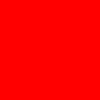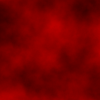## Procedural Generation Challenge

Thanks to a project I'm doing at work I started reading a book on procedural content generation. ProcGen (as apparently the cool kids call it) is all about creating interesting systems with randomness, which inevitably brings up texture generation, which brings up Perlin Noise, one of the most useful sources of randomness.

I've studied Perlin Noise before but I've always have trouble grasping it intuitively. I've learned how to calculate it by following tutorials, but honestly the actual noise generation is the least interesting part of it.

The tricky part is getting an intuitive sense of how to use noise. If you've ever used Quartz Composer or other node based systems (meaning a boxes and line graph interface, not NodeJS) then you've seen how they work. You wire up a bunch of blocks then endlessly tweak the variables. But how do the variables effect the output? If you are trying to get a certain look, then how should you adjust the variables? I don't know. I lack an intuition of how the equations combine, so that I can have a base to start with. It's like paint. I may not know where each bristle will land exactly, but I have an intuitive sense of how the paint will flow and combine based on my experience with paint. I need that experience with noise functions.

So.. I'm going to try an experiment. Taking inspiration from SigHack I'm going to try to generate 25 output textures. 25 different textures. As different as possible. This will force me to try different techniques; and hopefully teaching me the intuition I seek. I'm also going to do it from scratch in code rather than using a visual tool, forcing me to truly understand how the equations work.

Will this be a success or will I end up with 25 Jackson Pollacks (to be clear, I'm not a fan of Jackson Pollack. It's just rainbow noise to me.) To force myself to do it, I'm going to blog about every step, hopefully revealing to both you and me an intuitive sense of how these things work. So let's get started.

### Getting Started

Rather than drawing in the browser I'm going just do it from the command line with NodeJS. Just me and a text editor, the way God intended. However, I do need to ability to generate images and save them to disk, so I'm opening up a new file with these imports.

``const pureimage = require('pureimage');const fs = require('fs');``

The `pureimage` library is a headless graphics API I wrote. It implements the HTML canvas API, but into a buffer. It has no native dependencies either. It just writes into memory. It can save to disk using the builtin `fs` package, so we'll need that as well.

Now let's create an empty array of data. I created a function called `gen` which turns a width and height into an array of arrays. Of course that requires a lot of annoying for loops, so let's bundle that up into a function.

``function gen(width, height) {    const rows = [];    for (let y = 0; y < height; y++) {        const row = [];        for(let x=0; x<width; x++) {            row[x] = 0;        }        rows[y] = row;    }    return rows;}``

To fill in the data we probably want to run a function on every pixel to produce a new pixel. That means more for loops, so let's create a function called `map()` which will take a callback and invoke it with the current xy in both pixel coordinates and uv space (meaning 0->1). It returns a new image without mutating the original.

``function map(data,cb) {    const width = data.length;    const height = data.length;    return data.map((row,py)=>{        return row.map((val,px) => {            const nx = px/width, ny = py/height;            return cb(val,x,y,nx,ny);        })    });}``

And of course we'll want to save our image to disk so we can actually look at it. I'll spare you the details of how the `pureimage` and `fs` modules work. We'll just make a nice function called `save` to do it.

``function save(data,name) {    const h = data.length    const w = data.length    const img = pureimage.make(w,h)    const c = img.getContext('2d')    c.fillStyle = 'white'    c.fillRect(0, 0, 10, 10)    map(data, (v,x,y)=>{        img.setPixelRGBA_i(x, y, v.r*255,                      v.g*255, v.b*255, 255);    });    pureimage.encodePNGToStream(img,                fs.createWriteStream(name))        .then(() => console.log("wrote",name));}``

Assuming everything works as designed, we can now create an empty image, fill it with the color red, and save it to disk like this:

``    const img = gen(100,100)    const img2 = map(img,(cur, px,py,ix,iy)=>{        return { r:1, g:0, b:0}    })    save(img2,'demo1.png')``

or, if we want to be very compact and lispy:

``save(map(gen(100,100), (cur, px,py,ix,iy)     => ({r:1,g:0,b:0})), 'demo2.png')``

which gives us this image.Fantastic!

Alright, now we can get some work done.

Next we need a source of noise. Since the actual noise generation function is both boring and tricky to get right, I'm going to use the `simplex-noise` library. I'll also create a function to generate noise in the range of 0 to 1, since that works better for doing graphics.

``const SimplexNoise = require('simplex-noise');let gen = new SimplexNoise();function snoise(nx, ny) {    // Rescale from -1.0:+1.0 to 0.0:1.0    return gen.noise2D(nx, ny) / 2 + 0.5;}``

If you've seen noise before you've probably seen things like displacement clouds or other foggy textures. These aren't actually the raw noise. Instead they come from octaves of noise. This means that we stack a bunch noise layers at different scales together to produce a final noise output. Because we use different scales there will be both large and small details. The common way to do this is to double the scale with each layer, which is why they are called octaves. Again, the actual implementation isn't that interesting, the concept is what matters.

``function octave_noise(nx,ny,octaves) {    let val = 0;    let freq = 1;    let max = 0;    let amp = 1;    for(let i=0; i<octaves; i++) {        val += snoise(nx*freq,ny*freq)*amp;        max += amp;        amp /= 2;        freq  *= 2;    }    return val/max;}``

Great. Now let's render some noise:

``save(map(gen(100,100), (cur,px,py,ix,iy) => {    const v = octave(ix,iy,8)    return {r:v,g:v,b:v}}), 'noise1.png')``Awesome. We've got some real noise!

Okay, that's it for tonight. See you soon, when we mix in some sin waves.